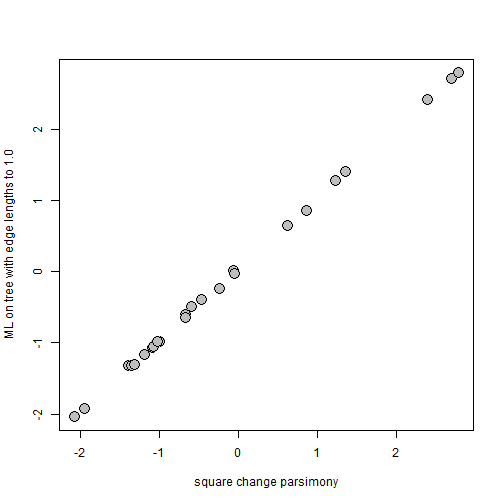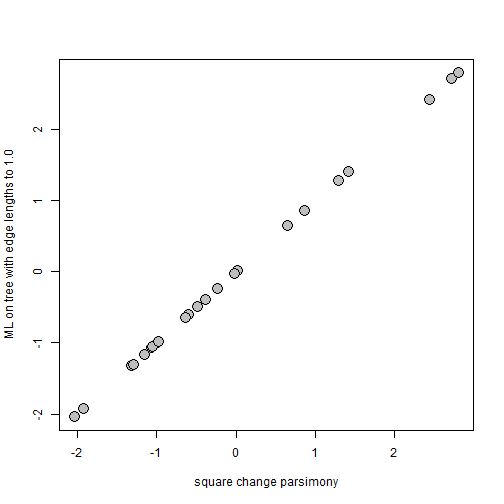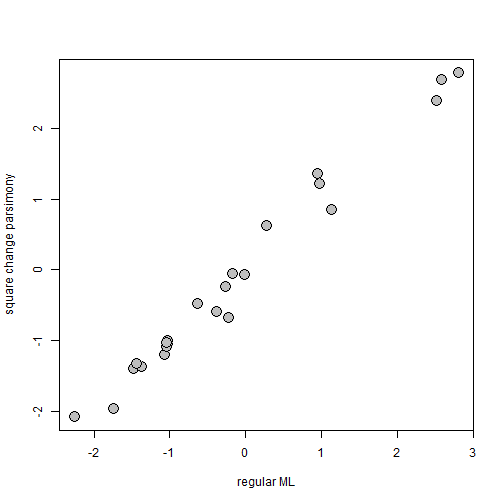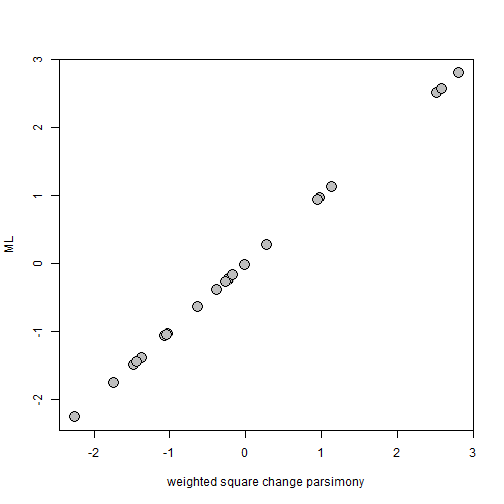## Thursday, January 31, 2019

### Unweighted & weighted square-change-parsimony vs. ML ancestral state estimation

A R-sig-phylo member today wrote:

“I want to get squared change parsimony ancestral state reconstructions for a matrix into R.

I have tried the `asr_max_parsimony()` function from castor, but the results are not the same as in Mesquite for example. To take an example, a two-taxon clade with a unique state for these taxa optimized as having a different state (shared by near relatives) at the ancestral node. This doesn't happen in Mesquite and is not what I want.

If there were some way to get the results from Mesquite into R that would also be OK, but I cannot figure out the node numbering system in Mesquite, to match them up with nodes as numbered by ape.”

My response to this is that square-change parsimony ancestral states (defined as the states that minimize the unweighted sum of square changes implied across all the edges of the tree) are the same as the ML ancestral states in which we set all edge lengths to the same (non-zero) value.

To demonstrate this, we can easily write our own simple square-change-parsimony function as follows:

``````square.change.parsimony<-function(tree,x,...){
if(hasArg(maxit)) maxit<-list(...)\$maxit
else maxit<-2000
if(hasArg(trace)) trace<-list(...)\$trace
else trace<-FALSE
if(hasArg(init)) init<-list(...)\$init
else init<-NULL
SSCH<-function(a,x,phy,trace){
A<-matrix(c(x[phy\$tip.label],
a[as.character(1:phy\$Nnode+Ntip(phy))])[phy\$edge],
nrow(phy\$edge),2)
SS<-sum((A[,2]-A[,1])^2)
if(trace) cat(paste("Sum of Squares :",round(SS,8),"\n"))
SS
}
if(is.null(init)) init<-fastAnc(tree,x)
fit<-optim(init,SSCH,x=x,phy=tree,trace=trace,
control=list(maxit=maxit))
object<-list(ace=unclass(fit\$par),sum.of.squares=fit\$value,
counts=fit\$counts,convergence=fit\$convergence,
message=fit\$message)
object
}
``````

Now we can compare it to ML values obtained (say) using `fastAnc`, but in which we first set all edge lengths of the tree to 1.0.

``````## simulate some data
library(phytools)
tree<-pbtree(n=26,tip.label=LETTERS)
x<-fastBM(tree)

## our square change parsimony states:
fit<-square.change.parsimony(tree,x,maxit=1e8) ## we have to set maxit to be quite high
fit
``````
``````## \$ace
##          27          28          29          30          31          32
## -0.58984343 -0.67455123 -0.06632310 -0.67313617 -0.05265349  0.86007564
##          33          34          35          36          37          38
## -1.95745914  1.22430357  1.36003054  0.62492090 -0.23894155  2.40076293
##          39          40          41          42          43          44
##  2.69448380  2.78748896 -1.39183963 -1.36220597 -2.07969284 -0.47162174
##          45          46          47          48          49          50
## -1.03879642 -1.19275392 -1.09041472 -1.08289504 -1.00470802 -1.02584958
##          51
## -1.32344518
##
## \$sum.of.squares
##  15.00555
##
## \$counts
##     7590       NA
##
## \$convergence
##  0
##
## \$message
## NULL
``````
``````## our ML states with all edges to 1.0:
utree<-tree
utree\$edge.length<-rep(1,nrow(tree\$edge))
sch<-fastAnc(utree,x)
sch
``````
``````## Ancestral character estimates using fastAnc:
##        27        28        29        30        31        32        33
## -0.491222 -0.597435  0.017300 -0.640381 -0.019996  0.868693 -1.918447
##        34        35        36        37        38        39        40
##  1.289716  1.417875  0.649351 -0.236074  2.433972  2.716463  2.802910
##        41        42        43        44        45        46        47
## -1.318385 -1.319005 -2.038713 -0.385008 -0.987479 -1.155175 -1.064055
##        48        49        50        51
## -1.050179 -0.978863 -0.983751 -1.297779
``````
``````## compare them
plot(fit\$ace,sch,pch=21,bg="grey",cex=2,xlab="square change parsimony",
ylab="ML on tree with edge lengths to 1.0")
``````If they're different, it may just be because we haven't converged on the genuine minimum sum of squares solution. We could run our optimization longer, change our tolerance, or (best of all) start with a superior solution. Let's try the lattermost of these options:

``````fit2<-square.change.parsimony(tree,x,maxit=1e8,init=sch)
fit
``````
``````## \$ace
##          27          28          29          30          31          32
## -0.58984343 -0.67455123 -0.06632310 -0.67313617 -0.05265349  0.86007564
##          33          34          35          36          37          38
## -1.95745914  1.22430357  1.36003054  0.62492090 -0.23894155  2.40076293
##          39          40          41          42          43          44
##  2.69448380  2.78748896 -1.39183963 -1.36220597 -2.07969284 -0.47162174
##          45          46          47          48          49          50
## -1.03879642 -1.19275392 -1.09041472 -1.08289504 -1.00470802 -1.02584958
##          51
## -1.32344518
##
## \$sum.of.squares
##  15.00555
##
## \$counts
##     7590       NA
##
## \$convergence
##  0
##
## \$message
## NULL
``````
``````plot(fit2\$ace,sch,pch=21,bg="grey",cex=2,xlab="square change parsimony",
ylab="ML on tree with edge lengths to 1.0")
``````Now, as a point of reference, let's compare to our standard ML estimates:

``````plot(fastAnc(tree,x),fit\$ace,pch=21,bg="grey",cex=2,xlab="regular ML",
ylab="square change parsimony")
``````So, in other words, there is no need to for a special square-change-parsimony function as you should be able to just use `ace` or `fastAnc`, but with all edges set to unit length; and this also tells us something important about square-change-parsimony - that (in its unweighted form) it assumes that the amount of change between a reconstructed ancestral value and any tip depends only on the number of edges separating them - and not at all on the length of those edges.

Finally, if we adapt our previous function such that we divide each squared change by the corresponding edge length before summing them:

``````weighted.square.change.parsimony<-function(tree,x,...){
if(hasArg(maxit)) maxit<-list(...)\$maxit
else maxit<-2000
if(hasArg(trace)) trace<-list(...)\$trace
else trace<-FALSE
if(hasArg(init)) init<-list(...)\$init
else init<-NULL
SSCH<-function(a,x,phy,trace){
A<-matrix(c(x[phy\$tip.label],
a[as.character(1:phy\$Nnode+Ntip(phy))])[phy\$edge],
nrow(phy\$edge),2)
SS<-sum((A[,2]-A[,1])^2/phy\$edge.length)
if(trace) cat(paste("Sum of Squares :",round(SS,8),"\n"))
SS
}
if(is.null(init)) init<-fastAnc(tree,x)
fit<-optim(init,SSCH,x=x,phy=tree,trace=trace,
control=list(maxit=maxit))
object<-list(ace=unclass(fit\$par),sum.of.squares=fit\$value,
counts=fit\$counts,convergence=fit\$convergence,
message=fit\$message)
object
}
``````

an analysis that has been called weighted square-change-parsimony, it turns out that this is exactly the same as regular ML:

``````fit3<-weighted.square.change.parsimony(tree,x)
plot(fit3\$ace,fastAnc(tree,x),pch=21,bg="grey",cex=2,
xlab="weighted square change parsimony",ylab="ML")
``````That's it.

#### 1 comment:

1.Hi, I was just also trying to compare methods. I found useful the myResult\$logLik data in the anc.ML function. However I am not sure how to calculate AIC. I would like to be able to use something like AIC(myResult) as the Rphylopars package is able to do.

Note: due to the very large amount of spam, all comments are now automatically submitted for moderation.